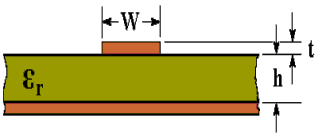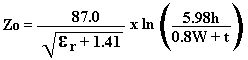# Microstrip Transmission Line Calculator Using IPC-2141 Equation

The characteristic impedance (Zo) of a microstrip transmission line can be calculated using the active calculator or the formula at the bottom of the page.INPUT DATA Relative Dielectric Constant ( εr ): Track Width: mm Track Thickness: mm Dielectric Thickness: mm
 RESULT Characteristic Impedance (Zo): Ohms

This calculator uses JavaScript and will function in most modern browsers. For more information see About our calculators

The calculator gives very similar results to those in Table 1 of an article at  http://www.polarinstruments.com/support/cits/IPC1999.pdf

The following formula is given in the above article by Polar Instruments.Where εr is the Relative Dielectric Constant

W is the Width of track

t is the Thickness of track (1 oz/ft2 = 0.035 mm)

h is the Thickness of dielectric

Note that the "h", for dielectric thickness in this formula is omitted in some other Polar publications.

The original source of formula is IPC-2141: Controlled Impedance Circuit Boards and High-Speed Logic Design, April 1996.

IPC have their website at: http://www.ipc.org/ContentPage.aspx?pageid=PCB-Tools-and-Calculators

This calculator is provided free by Chemandy Electronics in order to promote the FLEXI-BOX